Updating search results...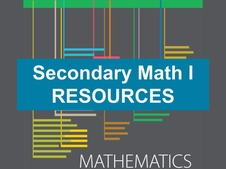# Secondary Mathematics I Resources

This collection contains highly recommended Secondary Mathematics I lessons, activities, and other resources from the eMedia library.

300 affiliated resources

# Search Resources

View
Selected filters: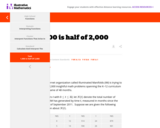Restricted Use
Rating
0.0 stars

This real-life modeling task could serve as a summative exercise in which many aspects of students' knowledge of functions are put to work.

Subject:
Mathematics
Material Type:
Activity/Lab
Provider:
Illustrative Mathematics
04/26/2022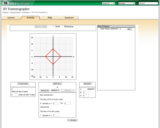Unrestricted Use
CC BY
Rating
0.0 stars

This lesson contains an applet that allows students to explore translations, reflections, and rotations.

Provider:
Interactive
06/22/2022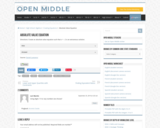Restricted Use
Rating
0.0 stars

Open Middle provides math problems that have a closed beginning, a closed end, and an open middle. This means that there are multiple ways to approach and ultimately solve the problems. Open middle problems generally require a higher Depth of Knowledge than most problems that assess procedural and conceptual understanding.

Subject:
Mathematics
Material Type:
Activity/Lab
Provider:
Open Middle Math
04/26/2022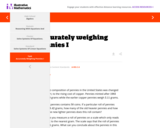Restricted Use
Rating
0.0 stars

This task asks students to solve systems of linear equations exactly and approximately (e.g., with graphs), focusing on pairs of linear equations in two variables.

Subject:
Mathematics
Material Type:
Activity/Lab
Provider:
Illustrative Mathematics
04/26/2022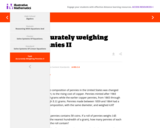Restricted Use
Rating
0.0 stars

This task is a somewhat more complicated version of ''Accurately weighing pennies I'' as a third equation is needed in order to solve part (a) explicitly.

Subject:
Mathematics
Material Type:
Activity/Lab
Provider:
Illustrative Mathematics
04/26/2022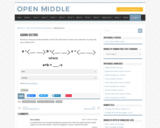Restricted Use
Rating
0.0 stars

Open Middle provides math problems that have a closed beginning, a closed end, and an open middle. This means that there are multiple ways to approach and ultimately solve the problems. Open middle problems generally require a higher Depth of Knowledge than most problems that assess procedural and conceptual understanding.

Subject:
Mathematics
Material Type:
Activity/Lab
Provider:
Open Middle Math
04/26/2022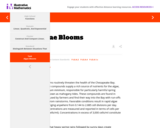Unrestricted Use
CC BY
Rating
0.0 stars

The problem statement describes a changing algae population as reported by the Maryland Department of Natural Resources. In part (a), students are expected to build an exponential function modeling algae concentration from the description given of the relationship between concentrations in cells/ml and days of rapid growth (F-LE.2). The intent of part (b) is for students to gain an appreciation for the exponential growth exhibited despite an apparently modest growth rate of 1 cell division per day.

Provider:
Illustrative Mathematics
06/22/2022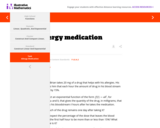Unrestricted Use
CC BY
Rating
0.0 stars

The purpose of the task is to help students become accustomed to evaluating exponential functions at non-integer inputs and interpreting the values.

Provider:
Illustrative Mathematics
06/22/2022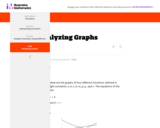Unrestricted Use
CC BY
Rating
0.0 stars

This task could be used as a review problem or as an assessment problem after many different types of functions have been discussed. Since the different parameters of the functions are not given explicitly, the focus is not just on graphing specific functions but rather students have to focus on how values of parameters are reflected in a graph.

Provider:
Illustrative Mathematics
06/22/2022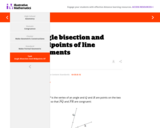Unrestricted Use
CC BY
Rating
0.0 stars

This task provides a construction of the angle bisector of an angle by reducing it to the bisection of an angle to finding the midpoint of a line segment.

Provider:
Illustrative Mathematics
06/22/2022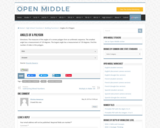Restricted Use
Rating
0.0 stars

Open Middle provides math problems that have a closed beginning, a closed end, and an open middle. This means that there are multiple ways to approach and ultimately solve the problems. Open middle problems generally require a higher Depth of Knowledge than most problems that assess procedural and conceptual understanding.

Subject:
Mathematics
Material Type:
Activity/Lab
Provider:
Open Middle Math
04/26/2022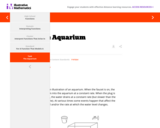Unrestricted Use
CC BY
Rating
0.0 stars

The purpose of this task is to connect graphs with real life situations. Graphs tell a story. Specific features of a graph connect to specific features of a story. A point on a graph captures a specific instant in the story.

Provider:
Illustrative Mathematics
06/22/2022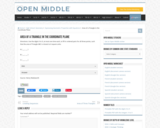Restricted Use
Rating
0.0 stars

Open Middle provides math problems that have a closed beginning, a closed end, and an open middle. This means that there are multiple ways to approach and ultimately solve the problems. Open middle problems generally require a higher Depth of Knowledge than most problems that assess procedural and conceptual understanding.

Subject:
Mathematics
Material Type:
Activity/Lab
Provider:
Open Middle Math
04/26/2022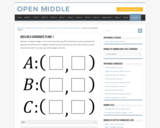Restricted Use
Rating
0.0 stars

Open Middle provides math problems that have a closed beginning, a closed end, and an open middle. This means that there are multiple ways to approach and ultimately solve the problems. Open middle problems generally require a higher Depth of Knowledge than most problems that assess procedural and conceptual understanding.

Subject:
Mathematics
Material Type:
Activity/Lab
Provider:
Open Middle Math
04/26/2022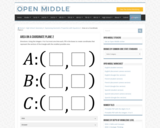Restricted Use
Rating
0.0 stars

Open Middle provides math problems that have a closed beginning, a closed end, and an open middle. This means that there are multiple ways to approach and ultimately solve the problems. Open middle problems generally require a higher Depth of Knowledge than most problems that assess procedural and conceptual understanding.

Subject:
Mathematics
Material Type:
Activity/Lab
Provider:
Open Middle Math
04/26/2022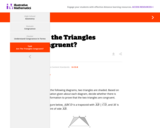Unrestricted Use
CC BY
Rating
0.0 stars

The purpose of this task is primarily assessment-oriented, asking students to demonstrate knowledge of how to determine the congruency of triangles.

Provider:
Illustrative Mathematics
06/22/2022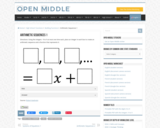Restricted Use
Rating
0.0 stars

Open Middle provides math problems that have a closed beginning, a closed end, and an open middle. This means that there are multiple ways to approach and ultimately solve the problems. Open middle problems generally require a higher Depth of Knowledge than most problems that assess procedural and conceptual understanding.

Subject:
Mathematics
Material Type:
Activity/Lab
Provider:
Open Middle Math
04/26/2022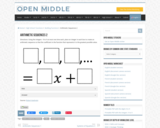Restricted Use
Rating
0.0 stars

Open Middle provides math problems that have a closed beginning, a closed end, and an open middle. This means that there are multiple ways to approach and ultimately solve the problems. Open middle problems generally require a higher Depth of Knowledge than most problems that assess procedural and conceptual understanding.

Subject:
Mathematics
Material Type:
Activity/Lab
Provider:
Open Middle Math
04/26/2022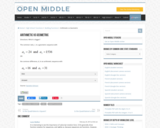Restricted Use
Rating
0.0 stars

Open Middle provides math problems that have a closed beginning, a closed end, and an open middle. This means that there are multiple ways to approach and ultimately solve the problems. Open middle problems generally require a higher Depth of Knowledge than most problems that assess procedural and conceptual understanding.

Subject:
Mathematics
Material Type:
Activity/Lab
Provider:
Open Middle Math
04/26/2022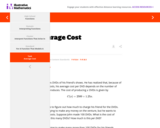Restricted Use